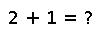# condskeel

condskeel(M, [x, p])

$$\kappa_S(M, p) & = \left\Vert \left\vert M \right\vert \left\vert M^{-1} \right\vert \right\Vert_p \ \kappa_S(M, x, p) & = \left\Vert \left\vert M \right\vert \left\vert M^{-1} \right\vert \left\vert x \right\vert \right\Vert_p$$

Skeel condition number $\kappa_S$ of the matrix M, optionally with respect to the vector x, as computed using the operator p-norm. p is Inf by default, if not provided. Valid values for p are 1, 2, or Inf.

This quantity is also known in the literature as the Bauer condition number, relative condition number, or componentwise relative condition number.

## Examples

Checking you are not a robot: